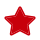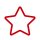Updated on 2022-11-09 GMT+08:00

# Rows Hints

#### Function

These hints specify the number of rows in an intermediate result set. Both absolute values and relative values are supported.

#### Syntax

 `1` ```rows(table_list #|+|-|* const) ```

#### Parameter Description

• #,+,-, and * are operators used for hinting the estimation. # indicates that the original estimation is used without any calculation. +,-, and * indicate that the original estimation is calculated using these operators. The minimum calculation result is 1. table_list specifies the tables to be joined. The values are the same as those of table_list in Join Operation Hints.
• const can be any non-negative number and supports scientific notation.

For example:

rows(t1 #5): The result set of t1 is five rows.

rows(t1 t2 t3 *1000): Multiply the result set of joined t1, t2, and t3 by 1000.

#### Suggestion

• The hint using * for two tables is recommended, because this hint will take effect for a join as long as the two tables appear on both sides of this join. For example, if the hint is rows(t1 t2 * 3), the join result of (t1 t3 t4) and (t2 t5 t6) will be multiplied by 3 because t1 and t2 appear on both sides of the join.
• rows hints can be specified for the result sets of a single table, multiple tables, function tables, and subquery scan tables.

#### Examples

Hint the query plan in Examples as follows:

 ```1 2``` ```explain select /*+ rows(store_sales store_returns *50) */ i_product_name product_name ... ```

Multiply the result set of joined store_sales and store_returns by 50. The optimized plan is as follows:The estimation value after the hint in row 11 is 360, and the original value is rounded off to 7.